Vol 17, No 7 (2017) / Chan

Can cold dark matter paradigm explain the central-surface-densities relation?

Can cold dark matter paradigm explain the central-surface-densities relation?

Chan Man-Ho

Department of Science and Environmental Studies, The Education University of Hong Kong, Tai Po, New Territories, Hong Kong, China

† Corresponding author. E-mail: chanmh@eduhk.hk

Abstract: Abstract

Recently, a very strong correlation between the central surface density of stars and dynamical mass in 135 disk galaxies has been obtained. It has been shown that this central-surface-densities relation agrees very well with Modified Newtonian Dynamics (MOND). In this article, we show that if we assume the baryons have an isothermal distribution and dark matter exists, then it is possible to derive by means of the Jeans equation an analytic central-surface-densities relation connecting dark matter and baryons that agrees with the observed relation. We find that the observed central-surface-densities relation can also be accommodated in the context of dark matter provided the latter is described by an isothermal profile. Therefore, the observed relation is consistent with not only MOND.

Keywords: (cosmology:) dark matter

1 Introduction

Recent empirical fits indicate a very strong correlation between the central surface density of stars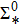and dynamical mass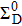in 135 disk galaxies (Lelli et al. ). The central-surface-densities relation can be described by a double power law (Lelli et al. )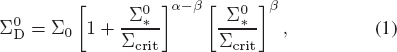where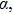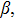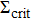and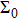are fitted parameters. Generally speaking, high surface brightness galaxies give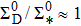while low surface brightness galaxies systematically deviate from unity. The observed scatter is small overall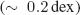and largely driven by observational uncertainties (Lelli et al. ). This result is surprising because there is no obvious reason why the central surface density of stars strongly correlates with the dynamical mass.

Recently, Milgrom () applied Modified Newtonian Dynamics (MOND) to derive this relation analytically. By using quasilinear MOND (QUMOND), we can obtain the expression in Milgrom ()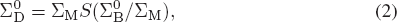where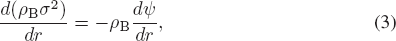where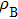is the baryonic mass density,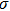is the velocity dispersion of baryonic matter and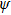is the total gravitational potential (including baryonic matter and dark matter). Note that the Jeans equation used here assumes isotropy and spherical symmetry. Nevertheless, it can also be applied in spiral galaxies if we only focus on the cylindrical radial direction in the galactic plane. The velocity dispersion would be directly proportional to the rotational velocity (Croton ). Since the isothermal distribution of baryons corresponds to the constant velocity dispersion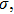by Equation (3), we get (Chan ; Evans et al. )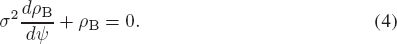By substituting the solution of the above equation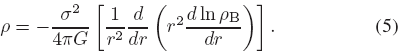Let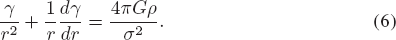Since the isothermal baryonic component yields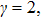we derive (Chan )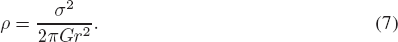This shows that if the baryonic component follows the isothermal distribution, the resulting total matter density also follows the isothermal distribution, which exhibits excellent agreement with observational data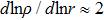(Koopmans et al. ; Velander et al. ; Grillo ). Note that we did not assume any isothermality of dark matter in the first place. We only assume baryons follow an isothermal distribution. Finally, based on the above derivation, the total potential that can yield the isothermal distribution of baryons is also isothermal. However, astrophysicists usually assume that dark matter follows the Navarro-Frenk-White (NFW) profile (Navarro et al. ), but not an isothermal profile. Even for some CDM simulations with baryons, no isothermal profile would be generated. Most of the resultant profiles are still an NFW profile, but with a shallower slope near the center (Schaller et al. ; Chan et al. ). This is because all of the simulations do not assume an isothermal distribution of baryons. Feedback can substantially change the stellar and dark matter dynamics and shape, causing the complete system to depart from a simple isothermal profile. This may be a reason why most CDM simulations do not generate an isothermal spherical distribution. In other words, if the collision between the baryonic matter is vigorous enough that the baryonic distribution can keep the isothermal distribution, the dark matter density and the total density also follow an isothermal profile. This is a result of solving the Jeans equation.

In addition, the above result can also give a simple explanation to the 'Halo-disk conspiracy problem' (why the transition from disk to halo domination is so smooth) (Battaner & Florido ; Remus et al. ). Since the total mass density consists of two components, the baryonic matter densityand dark matter density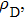we can write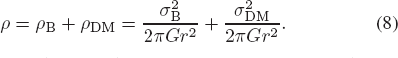Here, we have used two parameters to represent the velocity dispersion of baryonic matter (i.e.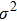. For a baryon dominated galaxy, we have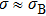. For a dark matter dominated galaxy, we have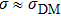Note that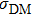is not the velocity dispersion of dark matter particles. The value ofis the limit for the velocity dispersion of baryonic matter in a dark matter dominated galaxy. On the other hand, since the observational data in galaxies strongly support the existence of a core in the dark matter density profile (de Blok ), we may slightly modify the dark matter density profile without destroying the isothermal distribution at largeby a cored-isothermal profile (Chan )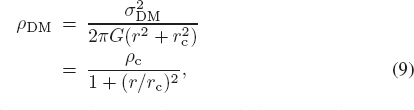where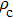and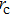are the central density and core radius of the dark matter profile respectively. The origin of a dark matter core (size ∼ Kpc) may be due to the self-interaction between dark matter particles (Spergel & Steinhardt ; Vogelsberger et al. ; Chan ) or some baryonic feedback such as supernovae (de Blok ; Governato et al. ). In fact, the existence of a dark matter core is still a controversial issue. Some studies claim that cores do not exist in galaxies (Fattahi et al. ). However, most observational data seem to favor the existence of dark matter cores (for a review, please see de Blok ; Bull et al. ; Del Popolo & Le Delliou ). Therefore, our model basically follows this assumption. Since the Jeans equation only describes the distribution of matter due to gravitational interaction, the effect of core formation due to other mechanisms at smallis not included. The small modification of the profile here is necessary to match the assumption of the existence of dark matter cores.

Recent studies suggest that the product of the central density and core radius of dark matter is almost a constant for many galaxies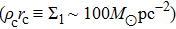(Gentile et al. ; Burkert ). Therefore, we can write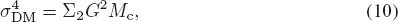where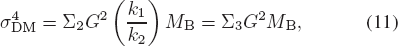where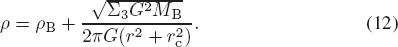Using the definition of the dynamical central surface density (Milgrom ), we get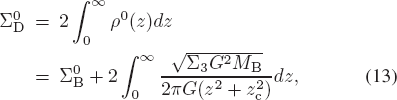where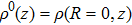is the central total mass density. Here, we work in cylindrical coordinates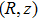with the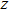-axis along the axisymmetry axis, and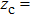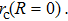By integrating the above equation, we get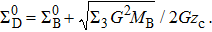Let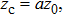where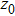is the characteristic disk length such that the Newtonian gravitational acceleration due to baryons just outside the disk is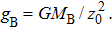Finally, we get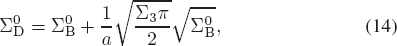where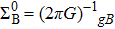in Milgrom (). Based on the derivation in QUMOND,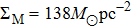for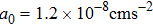(Milgrom ). Taking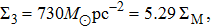we can write the above equation in terms of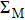Since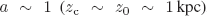we have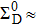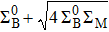. For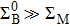(baryonic matter dominates the galaxy), we have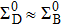The opposite asymptote (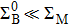) gives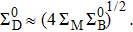These asymptotic results are identical to the results in (Milgrom ). The only difference is the functional form. Milgrom's result gives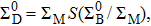where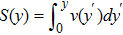(Milgrom ).

In Figure 1, we fit our result with the observed data obtained from Lelli et al. () and compare with Milgrom's result. Here, we assume that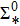is the proxy for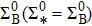(Milgrom ). Both theories can give the same agreement with observational data (our model with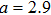gives a better fit). Therefore, we conclude that the observational data also support the dark matter paradigm if dark matter has an isothermal distribution, but not only using MOND theory.

 2016 ). The units of the central surface densities are in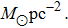'>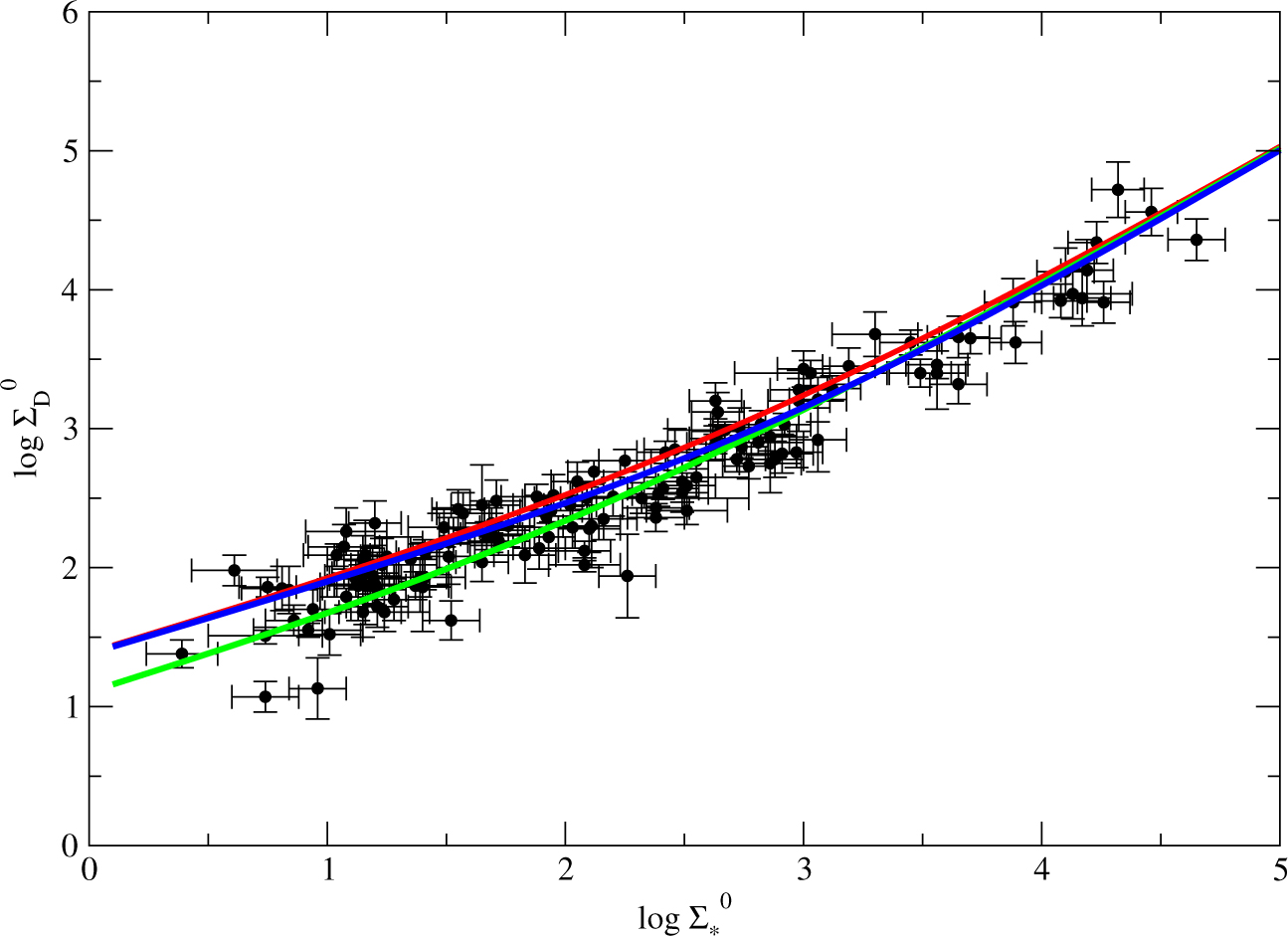Fig. 1 The resulting central-surface-densities relation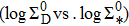and the observed data with error bars (Lelli et al. ). Here, we assume thatis the proxy for(Milgrom ). Red solid line: the relation in Eq. (15) with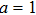Green solid line: the relation in Eq. (15) withBlue solid line: Milgrom's relation (Milgrom ). The units of the central surface densities are in3 Discussion

In this article, we derive the central-surface-densities relation by using the steady-state Jeans equation in the dark matter framework. If the baryonic density distribution is isothermal, the resultant total mass density also follows an isothermal distribution. This result agrees with the observational data and explains why rotational curves are flat for many galaxies (Sofue & Rubin ). We also relate the dark matter density profile with the baryonic matter content and show that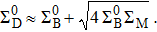The asymptotic relations for both regimes are identical to Milgrom's result. Milgrom () claims that there is no reason why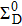is so well correlated with local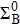in the dark matter paradigm. However, as shown in our derivation, the existence of a dark matter core may give a reason why these quantities are correlated. Therefore, the claim in Milgrom () is wrong. Generally speaking, both MOND and dark matter paradigms can give the same agreement with the observed central-surface-densities relation. In fact, some recent studies also show a similar conclusion by using CDM models (Di Cintio & Lelli ; Navarro et al. ).

In the derivation, there are a few constants involved: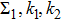and a. Although these values are not universal constants for all galaxies, the ranges of these values are quite narrow (Gentile et al. ; Rocha et al. ; Kassin et al. ). For example, the value of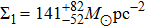is nearly a constant for a luminosity range of 14 magnitudes and the whole Hubble sequence (Gentile et al. ). The variations in these constants among different galaxies would contribute to the scatter in the resulting relation.

However, as mentioned in the introduction, MOND works very poorly in galaxy clusters. Most predictions for galaxy clusters in MOND theory do not match the observational data, including the lensing results (Ferreras et al. ). Although the failure of MOND on large scale is not related to our discussion here, MOND, suggested to be a universal theory, should work on all scales. Therefore, it is reasonable to suspect that MOND working well in galaxies is just a coincidence. If the observational data in galaxies support both paradigms but MOND does not work for galaxy clusters, it is reasonable to deny MOND is an effective theory to explain the missing mass problem. Moreover, Chan () shows that MOND is equivalent to a particular form of dark matter density profile (isothermal distribution) in the dark matter model. It also explains why MOND works in galaxies but not in galaxy clusters (Chan ). Although many studies have shown that the CDM model predictions for small scale structures (e.g. in dwarf galaxies) do not agree with observations (de Blok ), recent studies have started to realize that baryonic feedback might be an important mechanism to reconcile the discrepancies between theory and observations (Macciò et al. ; Peñarrubia et al. ; Pontzen & Governato ). Our result basically supports this argument. Provided the dark matter and baryon distributions are described by an isothermal sphere profile, the dark matter can also accommodate the missing mass problem. It is therefore not essential to invoke new physics (MOND) to address the current missing mass problem.

 Angus G.W. 2009 MNRAS 394 527 Battaner E. Florido E. 2000 Fund. Cosmic Phys. 21 1 Boylan-Kolchin M. Bullock J.S. Kaplinghat M. 2011 MNRAS 415 L40 Bull P. Akrami Y. Adamek J. et al. 2016 Physics of the Dark Universe 12 56 Burkert A. 2015 ApJ 808 158 Chan M.H. 2013a Phys. Rev. D 88 103501 Chan M.H. 2013b MNRAS 433 2310 Chan T.K. Kereš D. Oñorbe J. et al. 2015 MNRAS 454 2981 Croton D.J. 2009 MNRAS 394 1109 de Blok W.J.G. 2010 Advances in Astronomy 2010 789293 Del Popolo A. Le Delliou M. 2017 Galaxies 5 17 Di Cintio A. Lelli F. 2016 MNRAS 456 L127 Dunkel J. 2004 ApJ 604 L37 Evans N.W. An J. Walker M.G. 2009 MNRAS 393 L50 Fattahi A. Navarro J.F. Sawala T. et al. 2016 arXiv:1607.06479 Ferreras I. Mavromatos N.E. Sakellariadou M. Yusaf M.F. 2012 Phys. Rev. D 86 083507 Gentile G. Famaey B. Zhao H. Salucci P. 2009 Nature 461 627 Governato F. Zolotov A. Pontzen A. et al. 2012 MNRAS 422 1231 Grillo C. 2012 ApJ 747 L15 Kaplinghat M. Turner M. 2002 ApJ 569 L19 Kassin S.A. de Jong R.S. Weiner B.J. 2006 ApJ 643 804 Koopmans L.V.E. Bolton A. Treu T. et al. 2009 ApJ 703 L51 Lelli F. McGaugh S.S. Schombert J.M. Pawlowski M.S. 2016 ApJ 827 L19 Macciò A.V. Stinson G. Brook C.B. et al. 2012 ApJ 744 L9 McGaugh S.S. 2005 ApJ 632 859 McGaugh S.S. 2012 AJ 143 40 Milgrom M. 1983 ApJ 270 365 Milgrom M. 2016 Physical Review Letters 117 141101 Natarajan P. Zhao H. 2008 MNRAS 389 250 Navarro J.F. Benítez-Llambay A. Fattahi A. et al. 2016 arXiv:1612.06329 Navarro J.F. Frenk C.S. White S.D.M. 1997 ApJ 490 493 Peñarrubia J. Pontzen A. Walker M.G. Koposov S.E. 2012 ApJ 759 L42 Pontzen A. Governato F. 2014 Nature 506 171 Remus R.-S. Burkert A. Dolag K. et al. 2013 ApJ 766 71 Rocha M. Peter A.H.G. Bullock J.S. et al. 2013 MNRAS 430 81 Sanders R.H. 1999 ApJ 512 L23 Sanders R.H. McGaugh S.S. 2002 ARA&A 40 263 Schaller M. Frenk C.S. Bower R.G. et al. 2015 MNRAS 451 1247 Sofue Y. Rubin V. 2001 ARA&A 39 137 Spergel D.N. Steinhardt P.J. 2000 Physical Review Letters 84 3760 Velander M. Kuijken K. Schrabback T. 2011 MNRAS 412 2665 Vogelsberger M. Zavala J. Loeb A. 2012 MNRAS 423 3740
Cite this article: Chan Man-Ho. Can cold dark matter paradigm explain the central-surface-densities relation?. Res. Astron. Astrophys. 2017; 7:074.

Refbacks

• There are currently no refbacks.